$\newcommand{\NN}{\mathbb{N}} \newcommand{\CC}{\mathbb{C}} \newcommand{\GG}{\mathbb{G}} \newcommand{\LL}{\mathbb{L}} \newcommand{\PP}{\mathbb{P}} \newcommand{\QQ}{\mathbb{Q}} \newcommand{\RR}{\mathbb{R}} \newcommand{\VV}{\mathbb{V}} \newcommand{\ZZ}{\mathbb{Z}} \newcommand{\FF}{\mathbb{F}} \newcommand{\KK}{\mathbb{K}} \newcommand{\UU}{\mathbb{U}} \newcommand{\EE}{\mathbb{E}} \newcommand{\Aa}{\mathcal{A}} \newcommand{\Bb}{\mathcal{B}} \newcommand{\Cc}{\mathcal{C}} \newcommand{\Dd}{\mathcal{D}} \newcommand{\Ee}{\mathcal{E}} \newcommand{\Ff}{\mathcal{F}} \newcommand{\Gg}{\mathcal{G}} \newcommand{\Hh}{\mathcal{H}} \newcommand{\Ii}{\mathcal{I}} \newcommand{\Jj}{\mathcal{J}} \newcommand{\Kk}{\mathcal{K}} \newcommand{\Ll}{\mathcal{L}} \newcommand{\Mm}{\mathcal{M}} \newcommand{\Nn}{\mathcal{N}} \newcommand{\Oo}{\mathcal{O}} \newcommand{\Pp}{\mathcal{P}} \newcommand{\Qq}{\mathcal{Q}} \newcommand{\Rr}{\mathcal{R}} \newcommand{\Ss}{\mathcal{S}} \newcommand{\Tt}{\mathcal{T}} \newcommand{\Uu}{\mathcal{U}} \newcommand{\Vv}{\mathcal{V}} \newcommand{\Ww}{\mathcal{W}} \newcommand{\Xx}{\mathcal{X}} \newcommand{\Yy}{\mathcal{Y}} \newcommand{\Zz}{\mathcal{Z}} \newcommand{\al}{\alpha} \newcommand{\la}{\lambda} \newcommand{\ga}{\gamma} \newcommand{\Ga}{\Gamma} \newcommand{\La}{\Lambda} \newcommand{\Si}{\Sigma} \newcommand{\si}{\sigma} \newcommand{\be}{\beta} \newcommand{\de}{\delta} \newcommand{\De}{\Delta} \renewcommand{\phi}{\varphi} \renewcommand{\th}{\theta} \newcommand{\om}{\omega} \newcommand{\Om}{\Omega} \renewcommand{\epsilon}{\varepsilon} \newcommand{\Calpha}{\mathrm{C}^\al} \newcommand{\Cbeta}{\mathrm{C}^\be} \newcommand{\Cal}{\text{C}^\al} \newcommand{\Cdeux}{\text{C}^{2}} \newcommand{\Cun}{\text{C}^{1}} \newcommand{\Calt}{\text{C}^{#1}} \newcommand{\lun}{\ell^1} \newcommand{\ldeux}{\ell^2} \newcommand{\linf}{\ell^\infty} \newcommand{\ldeuxj}{{\ldeux_j}} \newcommand{\Lun}{\text{\upshape L}^1} \newcommand{\Ldeux}{\text{\upshape L}^2} \newcommand{\Lp}{\text{\upshape L}^p} \newcommand{\Lq}{\text{\upshape L}^q} \newcommand{\Linf}{\text{\upshape L}^\infty} \newcommand{\lzero}{\ell^0} \newcommand{\lp}{\ell^p} \renewcommand{\d}{\ins{d}} \newcommand{\Grad}{\text{Grad}} \newcommand{\grad}{\text{grad}} \renewcommand{\div}{\text{div}} \newcommand{\diag}{\text{diag}} \newcommand{\pd}{ \frac{ \partial #1}{\partial #2} } \newcommand{\pdd}{ \frac{ \partial^2 #1}{\partial #2^2} } \newcommand{\dotp}{\langle #1,\,#2\rangle} \newcommand{\norm}{|\!| #1 |\!|} \newcommand{\normi}{\norm{#1}_{\infty}} \newcommand{\normu}{\norm{#1}_{1}} \newcommand{\normz}{\norm{#1}_{0}} \newcommand{\abs}{\vert #1 \vert} \newcommand{\argmin}{\text{argmin}} \newcommand{\argmax}{\text{argmax}} \newcommand{\uargmin}{\underset{#1}{\argmin}\;} \newcommand{\uargmax}{\underset{#1}{\argmax}\;} \newcommand{\umin}{\underset{#1}{\min}\;} \newcommand{\umax}{\underset{#1}{\max}\;} \newcommand{\pa}{\left( #1 \right)} \newcommand{\choice}{ \left\{ \begin{array}{l} #1 \end{array} \right. } \newcommand{\enscond}{ \left\{ #1 \;:\; #2 \right\} } \newcommand{\qandq}{ \quad \text{and} \quad } \newcommand{\qqandqq}{ \qquad \text{and} \qquad } \newcommand{\qifq}{ \quad \text{if} \quad } \newcommand{\qqifqq}{ \qquad \text{if} \qquad } \newcommand{\qwhereq}{ \quad \text{where} \quad } \newcommand{\qqwhereqq}{ \qquad \text{where} \qquad } \newcommand{\qwithq}{ \quad \text{with} \quad } \newcommand{\qqwithqq}{ \qquad \text{with} \qquad } \newcommand{\qforq}{ \quad \text{for} \quad } \newcommand{\qqforqq}{ \qquad \text{for} \qquad } \newcommand{\qqsinceqq}{ \qquad \text{since} \qquad } \newcommand{\qsinceq}{ \quad \text{since} \quad } \newcommand{\qarrq}{\quad\Longrightarrow\quad} \newcommand{\qqarrqq}{\quad\Longrightarrow\quad} \newcommand{\qiffq}{\quad\Longleftrightarrow\quad} \newcommand{\qqiffqq}{\qquad\Longleftrightarrow\qquad} \newcommand{\qsubjq}{ \quad \text{subject to} \quad } \newcommand{\qqsubjqq}{ \qquad \text{subject to} \qquad }$

1-D Haar Wavelets

# 1-D Haar Wavelets

This numerical tour explores 1-D multiresolution analysis with Haar transform. It was introduced in 1910 by Haar [Haar1910] and is arguably the first example of wavelet basis.

## Installing toolboxes and setting up the path.

You need to download the following files: signal toolbox and general toolbox.

You need to unzip these toolboxes in your working directory, so that you have toolbox_signal and toolbox_general in your directory.

For Scilab user: you must replace the Matlab comment '%' by its Scilab counterpart '//'.

Recommandation: You should create a text file named for instance numericaltour.sce (in Scilab) or numericaltour.m (in Matlab) to write all the Scilab/Matlab command you want to execute. Then, simply run exec('numericaltour.sce'); (in Scilab) or numericaltour; (in Matlab) to run the commands.

Execute this line only if you are using Matlab.

getd = @(p)path(p,path); % scilab users must *not* execute this


Then you can add the toolboxes to the path.

getd('toolbox_signal/');
getd('toolbox_general/');


## Forward Haar Transform

The Haar transform is the simplest orthogonal wavelet transform. It is computed by iterating difference and averaging between odd and even samples of the signal.

Size $$N$$ of the signal.

N = 512;


First we load a 1-D signal $$f \in \RR^N$$.

name = 'piece-regular';
f = rescale( load_signal(name, N) );


The Haar transform operates over $$J = \log_2(N)-1$$ scales. It computes a series of coarse scale and fine scale coefficients $$a_j, d_j \in \RR^{N_j}$$ where $$N_j=2^j$$.

J = log2(N)-1;


The forward Haar transform computed $$\Hh(f) = (d_j)_{j=0}^J \cup \{a_0\}$$. Note that the set of coarse scale coefficients $$(a_j)_{j>0}$$ are not stored.

This transform is orthogonal, meaning $$\Hh \circ \Hh^* = \text{Id}$$, and that there is the following conservation of energy $\sum_i \abs{f_i}^2 = \norm{f}^2 = \norm{\Hh f}^2 = \sum_j \norm{d_j}^2 + \abs{a_0}^2.$

One initilizes the algorithm with $$a_{J+1}=f$$. The set of coefficients $$d_{j},a_j$$ are computed from $$a_{j+1}$$ as $\forall k=0,\ldots,2^j-1, \quad a_j[k] = \frac{a_{j+1}[2k] + a_{j+1}[2k+1]}{\sqrt{2}} \qandq d_j[k] = \frac{a_{j+1}[2k] - a_{j+1}[2k+1]}{\sqrt{2}}.$

Shortcut to compute $$a_j, d_j$$ from $$a_{j+1}$$.

haar = @(a)[   a(1:2:length(a)) + a(2:2:length(a));
a(1:2:length(a)) - a(2:2:length(a)) ]/sqrt(2);


Display the result of the one step of the transform.

clf;
subplot(2,1,1);
plot(f); axis('tight'); title('Signal');
subplot(2,1,2);
plot(haar(f)); axis('tight'); title('Transformed');The output of the forward transform is stored in the variable fw. At a given iteration indexed by a scale j, it will store in fw(1:2^(j+1)) the variable $$a_{j+1}$$, and in the remaining entries the variable $$d_{j+1},d_{j+2},\ldots,d_J$$.

Initializes the algorithm at scale $$j=J$$.

j = J;


Initialize fw to the full signal.

fw = f;


At iteration indexed by $$j$$, select the sub-part of the signal containing $$a_{j+1}$$, and apply it the Haar operator.

fw(1:2^(j+1)) = haar(fw(1:2^(j+1)));


Display the signal and its coarse coefficients.

s = 400; t = 40;
clf;
subplot(2,1,1);
plot(f,'.-'); axis([s-t s+t 0 1]); title('Signal (zoom)');
subplot(2,1,2);
plot(fw(1:2^j),'.-'); axis([(s-t)/2 (s+t)/2 min(fw(1:2^j)) max(fw(1:2^j))]); title('Averages (zoom)');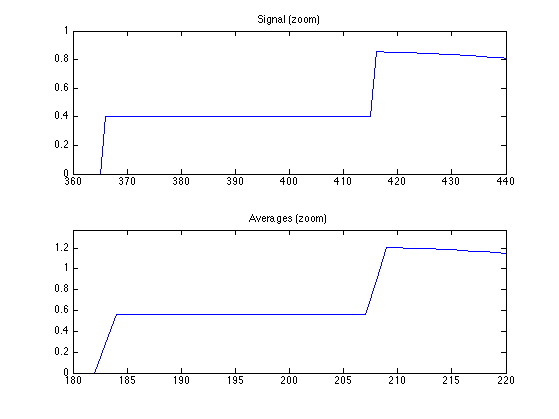Exercice 1: (check the solution) Implement a full wavelet Haar transform that extract iteratively wavelet coefficients, by repeating these steps. Take care of choosing the correct number of steps.

exo1;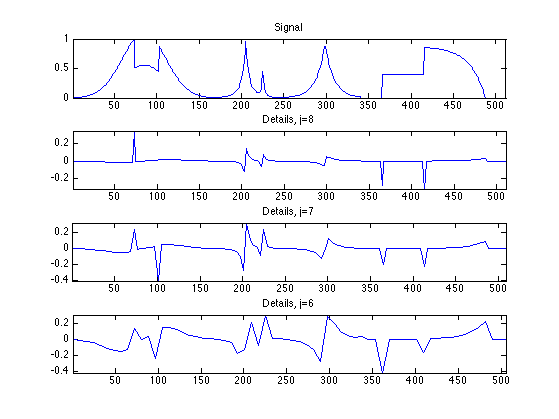Check that the transform is orthogonal, which means that the energy of the coefficient is the same as the energy of the signal.

fprintf('Should be 0: %.3f.\n', (norm(f)-norm(fw))/norm(f));

Should be 0: 0.000.


We display the whole set of coefficients fw, with red vertical separator between the scales. Can you recognize where are the low frequencies and the high frequencies?

clf;
plot_wavelet(fw);
axis([1 N -2 2]);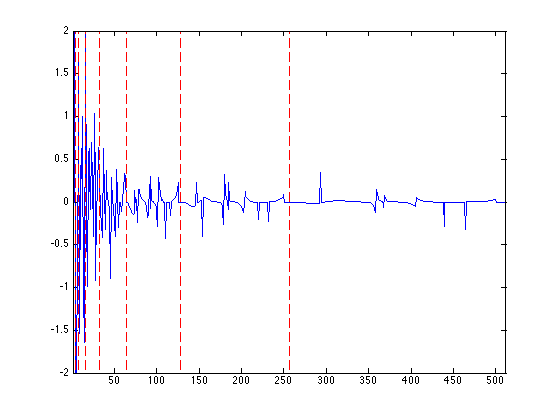## Backward Haar Transform

The backward transform computes a signal $$f_1 = \Hh^*(h)$$ from a set of coefficients $$h = (d_j)_{j=0}^J \cup \{a_0\}$$

If $$h = \Hh(f)$$ are the coefficients of a signal $$f$$, one recovers $$f_1 = f$$.

The inverse transform starts from $$j=0$$, and computes $$a_{j+1}$$ from the knowledge of $$a_j,d_j$$ as $\forall k = 0,\ldots,2^j-1, \quad a_{j+1}[2k] = \frac{ a_j[k] + d_j[k] }{\sqrt{2}} a_{j+1}[2k+1] = \frac{ a_j[k] - d_j[k] }{\sqrt{2}}$

One step of inverse transform

ihaar = @(a,d)assign( zeros(2*length(a),1), ...
[1:2:2*length(a), 2:2:2*length(a)], [a+d; a-d]/sqrt(2) );


Initialize the signal to recover $$f_1$$ as the transformed coefficient, and select the smallest possible scale $$j=0$$.

f1 = fw;
j = 0;


Apply one step of inverse transform.

f1(1:2^(j+1)) = ihaar(f1(1:2^j), f1(2^j+1:2^(j+1)));


Exercice 2: (check the solution) Write the inverse wavelet transform that computes f1 from the coefficients fw.

exo2;Check that we have correctly recovered the signal.

fprintf('Should be 0: %.3f.\n', (norm(f-f1))/norm(f));

Should be 0: 0.000.


## Haar Linear Approximation

Linear approximation is obtained by setting to zeros the Haar coefficient coefficients above a certain scale $$j$$.

Cut-off scale.

j = J-1;


Set to zero fine scale coefficients.

fw1 = fw; fw1(2^j+1:end) = 0;


Computing the signal $$f_1$$ corresponding to these coefficients fw1 computes the orthogonal projection on the space of signal that are constant on disjoint intervals of length $$N/2^j$$.

Exercice 3: (check the solution) Display the reconstructed signal obtained from fw1, for a decreasing cut-off scale $$j$$.

exo3;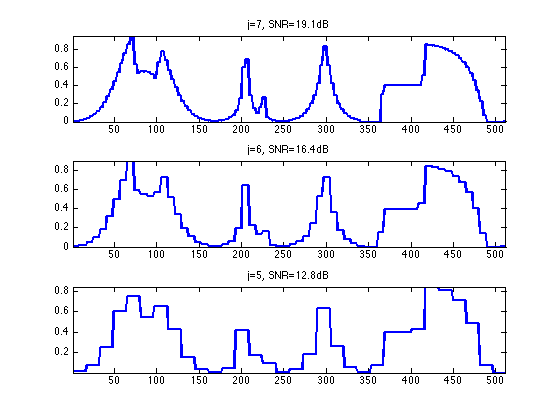## Haar Non-linear Approximation

Non-linear approximation is obtained by thresholding low amplitude wavelet coefficients. It is defined as $f_T = \Hh^* \circ S_T \circ \Hh(f)$ where $$S_T$$ is the hard tresholding operator $S_T(x)_i = \choice{ x_i \qifq \abs{x_i}>T, \\ 0 \quad \text{otherwise}. }.$

S = @(x,T) x .* (abs(x)>T);


Set the threshold value.

T = .5;


Threshold the coefficients.

fwT = S(fw,T);


Display the coefficients before and after thresholding.

clf;
subplot(2,1,1);
plot_wavelet(fw); axis('tight'); title('Original coefficients');
subplot(2,1,2);
plot_wavelet(fwT); axis('tight'); title('Thresholded coefficients');Exercice 4: (check the solution) Find the threshold $$T$$ so that the number of remaining coefficients in fwT is a fixed number $$m$$. Use this threshold to compute fwT and then display the corresponding approximation $$f_1$$ of $$f$$. Try for an increasing number $$m$$ of coeffiients.

exo4;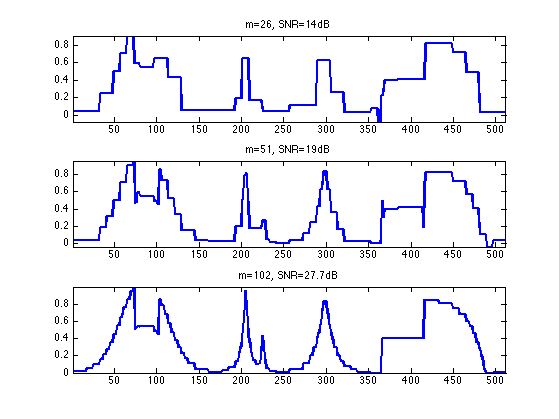## The Shape of a Wavelet

A wavelet coefficient corresponds to an inner product of $$f$$ with a wavelet Haar atom $$\psi_{j,k}$$ $d_j[k] = \dotp{f}{\psi_{j,k}}$

The wavelet $$\psi_{j,k}$$ can be computed by applying the inverse wavelet transform to fw where fw[m]=1 and fw[s]=0 for $$s \neq m$$ where $$m = 2^j+k$$.

Exercice 5: (check the solution) Compute wavelets at several positions and scales.

exo5;